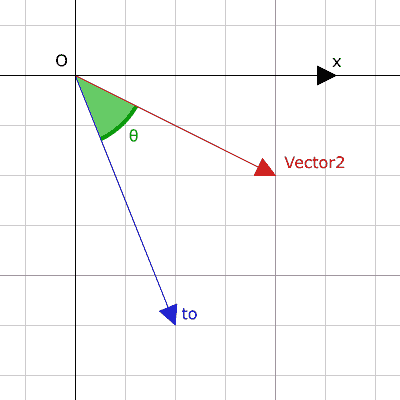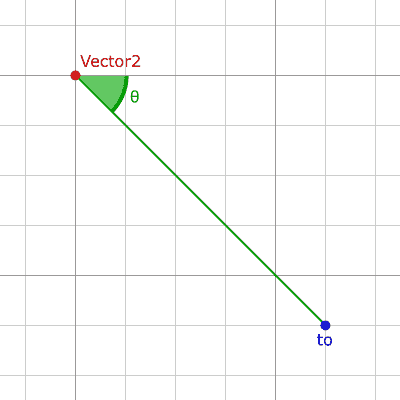I'm trying an alternative way to direct a shot in a football game using angleto rather than directionto:

Player.gd

``````var goal_target_position = ...

# current method:
var goal_direction = self.position.direction_to(goal_target_position)

# alternative method: calculate angle to target
var goal_target_position_angle = self.position.angle_to(goal_target_position)
var goal_direction_from_angle = Vector2.UP.rotated(goal_target_position_angle)

# these are different?
print("goal_direction: ", goal_direction)
print("goal_direction_from_angle: ", goal_direction_from_angle)
``````

..however, both goaldirection and goaldirectionfromangle are quite different and I don't know why. Can anyone spot what I might be doing wrong? Thanks

Godot version 3
in Engine

+1 vote

`angle_to` calculates the angle between two Vector2 assuming those vectors are lines, like this:Whereas `angle_to_point` calculates the angle between two Vector2 assuming those vectors are points in space, like this:So in your case, you probably want to use `angle_to_point` instead of `angle_to`.

by (21 points)

Thank you, works beautifully now!